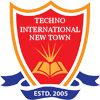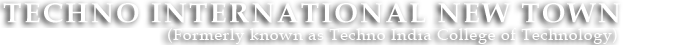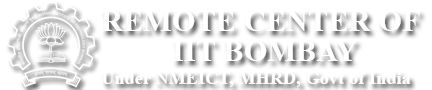#+91 9674112076/2079
••••# Basic Electrical Labortory-I & II (Lab-1)

ES-EE 191 (1st Semester)

Major Equipments:

•  R-L, R-C and R-L-C series and parallel compact kit.
•  R-L-C series and parallel circuit set up.
•  R-L-C series resonance frequency determination kit.
•  Single phase transformer set-up.
•  DC machine, Induction machine, Synchronous machine and single phase induction machine.
•  Three phase transformer.
•  Three phase circuit
•  Separately excited DC motor experiment set up.
•  DC-DC converter, DC-AC converter.
•  Components of LT switchgear.
•  Fluorescent Lamp,Heavy duty copper polyester choke,Starter,Variac.
•  Carbon lamp,Rheostat,Capacitor,Portable 1Ø Wattmeter.
•  1Ø Variac, Portable 1Ø Wattmeter (L.P.F), Tungsten Filament Lamp,Dynamometer type wattmeter.
•  Analog voltmeters,Analog Ammeters,Portable 1Ø Wattmeter (U.P.F).
•  Digital Voltmeters,Digital Ammeters, Multimeters, Oscilloscope, 3 Point Starter,Moving Iron Voltmeter.
•  DC voltage sources,Resistors,Variable resistors(Pot).
•  Superposition, Thevenin, Norton, Maximum Power Transfer Theorems’ test kits.

Experiment List:

•  First activity: Introduction to basic safety precautions and mentioning the do’s and Don’ts. Noting a list of experiments to be performed, and instructions for writing   the laboratory reports by the students. Group formation. Students are to be informed about the modalities of evaluation.
•  Introduction and uses of following instruments: (a) Voltmeter (b) Ammeter (c) Multimeter (d) Oscilloscope Demonstration of real life resistors, capacitors with color   code, inductors and autotransformer.
•  Demonstration of cut-out sections of machines: DC machine, Induction machine, Synchronous machine and single phase induction machine.
•  Calibration of ammeter and Wattmeter.
•  Determination of steady state and transient response of R-L, R-C and R-L-C circuit to a step change in voltage.
•  Determination of steady state response of R-L and R-C and R-L-C circuit and calculation of impedance and power factor.
•  Determination of resonance frequency and quality factor of series and parallel R-L-C circuit.
(a) Open circuit and short circuit test of a single-phase transformer.
(b) Load test of the transformer and determination of efficiency and regulation.
•  Demonstration of three phase transformer connections. Voltage and current relationship, phase shifts between the primary and secondary side.
•  Measurement of power in a three phase unbalanced circuit by two wattmeter method.
•  Determination of Torque –Speed characteristics of separately excited DC motor.
•  Determination of Torque speed characteristics and observation of direction reversal by change of phase sequence of connection of Induction motor.
•  Determination of operating characteristics of Synchronous generator.
•  Demonstration of operation of (a) DC-DC converter (b) DC-AC converter (c) DC-AC converter for speed control of an Induction motor
•  Demonstration of components of LT switchgear.

Beyond the Curriculum:

• Connection and measurement of power consumption of fluorescent lamps.
• V-I characteristics of carbon filament lamps.
•  Verification of the Thevenin's Theorem.
•  Verification of the Norton's Theorem.
•  Verification of the Superposition Theorem.
•  Verification of the Maximum Power Transfer Theorem.
•  Starting and Speed control of a DC shunt motor.
•  Calibration of M. I. type voltmeter and ammeter by DC Potentiometer